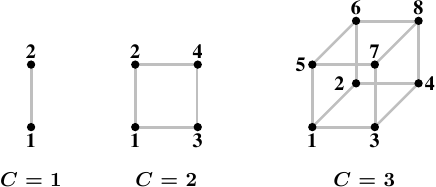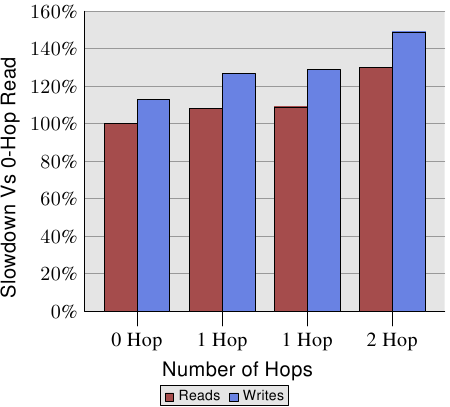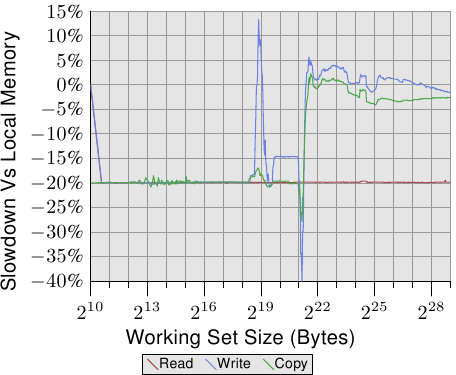2022/06/20

# 5 NUMA 支持

## 5.1 NUMA 硬件

NUMA还特别适用于大型机器。我们已经描述过了让多个处理器访问同一个内存的问题。对于商业硬件，所有处理器都将共享同一个北桥（暂时忽略AMD Opteron NUMA节点，它们有自己的问题）。这使得北桥成为一个严重的瓶颈，因为所有内存访问都是通过它路由的。当然，大型机器可以使用定制硬件代替北桥，但是，除非使用的内存芯片有多个端口，即它们可以从多个总线使用，否则仍然存在瓶颈。多端口RAM的构建和支持既复杂又昂贵，因此几乎从未使用过。图 5.1: Hypercubes

## 5.4 访问非本节点内存的开销图 5.3: 多节点之上的读写性能

00400000 default file=/bin/cat mapped=3 N3=3
00504000 default file=/bin/cat anon=1 dirty=1 mapped=2 N3=2
00506000 default heap anon=3 dirty=3 active=0 N3=3
38a9000000 default file=/lib64/ld-2.4.so mapped=22 mapmax=47 N1=22
38a9119000 default file=/lib64/ld-2.4.so anon=1 dirty=1 N3=1
38a911a000 default file=/lib64/ld-2.4.so anon=1 dirty=1 N3=1
38a9200000 default file=/lib64/libc-2.4.so mapped=53 mapmax=52 N1=51 N2=2
38a933f000 default file=/lib64/libc-2.4.so
38a943f000 default file=/lib64/libc-2.4.so anon=1 dirty=1 mapped=3 mapmax=32 N1=2 N3=1
38a9443000 default file=/lib64/libc-2.4.so anon=1 dirty=1 N3=1
38a9444000 default anon=4 dirty=4 active=0 N3=4
2b2bbcdce000 default anon=1 dirty=1 N3=1
2b2bbcde4000 default anon=2 dirty=2 N3=2
2b2bbcde6000 default file=/usr/lib/locale/locale-archive mapped=11 mapmax=8 N0=11
7fffedcc7000 default stack anon=2 dirty=2 N3=2


Figure 5.2: Content of /proc/PID/numa_mapsFigure 5.4: Operating on Remote Memory